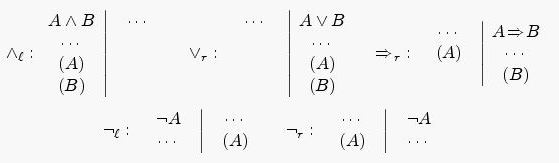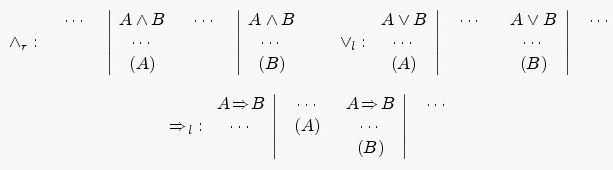Online JudgeProblem SetAuthorsOnline ContestsUser
Web Board
F.A.Qs
Statistical Charts
Problems
Submit Problem
Online Status
Prob.ID:
Register
Authors ranklist
Current Contest
Past Contests
Scheduled Contests
Award Contest
Register
Language:
Beth Tableaux
 Time Limit: 10000MS Memory Limit: 64000K Total Submissions: 211 Accepted: 37 Case Time Limit: 2000MS Special Judge

Description

Your program has to check the validity of a given logical formula. If the formula E is invalid (i.e. evaluates to 0 for some assignment of boolean values to the variables), you'll also have to find such an assignment of values.
Usually such tasks are solved just by processing all possible assignments of values to the variables occurring in E; if there are k such variables, this requires considering 2k such assignments. Of course, this is almost impossible for large values of k. Besides, this process is much different from the way people usually check (i.e. prove) logical formulae.
In this task you are to implement a natural way of checking validity of logical formulae based on the so-called Beth tableaux. This way of checking validity of formulae models to a certain extent the process of finding proofs or contrary instances used by humans, at least, a short rigorous proof can be usually obtained quite immediately from constructed Beth tableaux.
Let us fix the syntax of logical formulae considered:
• Constants 1 and 0;
• Variables --- letters from A to Z and from a to z;
• Parentheses --- if E is a formula, then (E) is another;
• Negation --- ￢E is a formula for any formula E;
• Conjunction --- E1∧E2∧ ... ∧En . Note that the conjunction is evaluated from left to right: E1∧E2∧E3 = (E1∧E2)∧E3.
• Disjunction --- E1∨E2∨ ... ∨En . The same remark applies.
• Implication --- E1 => E2 . Unlike the previous two operations it is evaluated from right to left:E1=>E2=>E3 means E1=>(E2 =>E3).
• Equivalence --- E1≡E2≡ ... ≡En . This expression is by definition computed as follows:(E1≡E2)∧(E2≡E3)∧ ... ∧(En-1≡En ).
The operations are listed from the highest priority to the lowest.
Let us define the principal operation of a compound formula E as the operation in E which is performed last (of course, formulae consisting just of one constant or variable have no principal operation). Also let us suppose that our formula does not contain equivalence operations since we can always replace E1≡E2 with (E1=>E2)∧(E2=>E1).
Now we define a Beth tableau to be a table with two columns with some formulae written in them. The order formulae are written in the columns and the number of times a formula appears in a column is irrelevant: we can assume for example that all formulae in a column are different and that they are ordered lexicographically.
Informally the left column corresponds to formulae which we want to be true and the right column to those which we want to be false; the whole process can be considered then as an attempt to produce a contrary instance to given formula E. A Beth tableau is said to be contradictory if some formula E* occurs in both columns of this tableau, or if 0 occurs in the left column, or if 1 occurs in the right column.
At every step of the process we have a collection of Beth tableaux. We start with a single Beth tableau with empty left column and the right column containing just the given formula E. A step of the process consists in choosing a compound formula C from a non-contradictory Beth tableau from the collection and applying a rule chosen by the principal operation of C and by the column (left or right) in which C is situated. As a result some formulae are added to the left or right columns of this Beth tableau (or both); of course, if these formulae that we want to add are already there the Beth tableau won't change, so in this case we say that the corresponding rule is inapplicable. There are also some rules that make two copies of the original Beth tableau and add some formulae into one of these copies and some other into the second one. In this case we say that the rule is inapplicable if both Beth tableaux thus obtained were already in the collection before applying the rule.
The process stops when no rule is applicable. Note that all formulae occurring in all Beth tableaux constructed will be sub-formulae of the original formula, so there are only finitely many Beth tableaux that can be obtained and thus only finitely many collections of Beth tableaux can be obtained. Therefore the process will have to stop at some point. If at this point all Beth tableaux in the collection are contradictory, the original formula E has been proved to be valid. Otherwise if τ is a non-contradictory Beth tableau from the collection, any variable x occurring in E must occur in exactly one column of τ (it cannot occur in both columns since τ is not contradictory; it must occur in at least one column since otherwise a rule would be applicable to the shortest formula in τ containing x). Then let us assign 1 to all the variables from the left column and 0 to the variables from the right column of τ . This would give us a contrary instance to E, i.e. an assignment of values to variables for which our original formula E evaluates to 0.
Now let us list all possible rules. It has been already explained that a rule is defined by the principal operation * of a formula C and the column (left or right) in which C was found. We shall label these rules by *l and *r where * is the operation (one of ∧, ∨, => or ￢). If * is binary, we shall assume that C = A * B; otherwise we assume C = ￢A.
Let us list the rules that do not increase the number of tableaux (i.e. they just add some formulae to the columns of selected Beth tableau):This means the following: for example, if we apply the rule =>r to the formula A=>B in the right column of a Beth tableau, then we have to add formula A to the left column and B to the right column of this tableau.
The next rules produce two Beth tableaux from one. A similar notation is used, but two Beth tableaux are shown for each rule:These three rules are interpreted as follows: if we apply for example ∨l to the formula A∨B in the left column of a Beth tableau τ, we have to replace τ with two copies of itselfτ' and τ'' , and then add A to the left column of τ' and B to the left column of τ'' .
• Input

The only line of input contains the formula represented as a string consisting of tokens '0', '1', 'A'. . . 'Z', 'a'. . . 'z', '(', ')', '~', '&', '|', '=>', '='. The last five tokens stand for ￢, ∧, ∨, => and ≡ respectively. Tokens can be separated by an arbitrary number of spaces. The line will contain at most 1 000 characters. The formula in the file will be syntactically correct.

Output

The output file must contain exactly one line. Output just "true" if the formula is valid or "false" followed by an assignment of variables that invalidates the formula. List the variables in lexicographical order. Adhere to the sample output as strictly as possible.

Sample Input

`K|~i|t|t|~e|~n`

Sample Output

`false: K=0, e=1, i=1, n=1, t=0`

Source

Northeastern Europe 2004, Northern Subregion

[Submit]   [Go Back]   [Status]   [Discuss]Home PageGo BackTo top